# Linear Regression Intro

If you see an error in the article, please comment or drop me an email.

# The basics of linear regression

Linear regression is one form of regression among others. It is probably the most intuitive and easiest one. The reason for regression is to 1) predict values for which there are no observed values and 2) to interprete and evaluate observed values themselves. As for the latter, it might be interesting to see which values are out of the ordinary.

When describing the association between two numerical variables, evaluate

• direction: positive (x,y), negative (x,y)

• form: linear or not

• strength: determined by the scatter around the underlying relationship

### Formula of the linear model

In linear regression, we try to estimate the following parameters: the slope beta_1 and the intercept beta_0.

`y_hat = b_0 + ( b_1 * x )`

• y_hat = the predicted value

• b_1 = the point estimate of the parameter beta_1 (slope)

• b_0 = the point estimate of the parameter beta_0 (the intercept)

How to find…

…b_1: `b_1 = (sd_result/sd_explanatory) * R`

…b_0: `b_0 = mean_response - (b_1 * mean_explanatory)`

…R: `R = (sd_result/sd_explanatory)/b_1`

…e: `e = response_var - b_0 - b_1 * explanatory_var`

### How to calculate the fit summary “by hand”

``````#Get the data
data(diamond)
y <- diamond\$price; x <- diamond\$carat;

n <- length(y)

beta1 <- cor(y, x) * sd(y) / sd(x) #the slope
beta0 <- mean(y) - beta1 * mean(x) #the intercept
e <- y - beta0 - beta1 * x #the residuals

sigma <- sqrt(sum(e^2) / (n-2)) #the residual standard deviation (=estimate of the variability around the regression line)
ssx <- sum((x - mean(x))^2) #sum of squares of x's (SST)
seBeta0 <- (1 / n + mean(x) ^ 2 / ssx) ^ .5 * sigma #standard error of the intercept
seBeta1 <- sigma / sqrt(ssx) #standard error of the slope

tBeta0 <- beta0 / seBeta0 # t statistic for the intercept
tBeta1 <- beta1 / seBeta1 # t statistic for the slope

pBeta0 <- 2 * pt(abs(tBeta0), df = n - 2, lower.tail = FALSE) #p-value
pBeta1 <- 2 * pt(abs(tBeta1), df = n - 2, lower.tail = FALSE) #p-value

#Show results
coefTable <- rbind(c(beta0, seBeta0, tBeta0, pBeta0), c(beta1, seBeta1, tBeta1, pBeta1))
colnames(coefTable) <- c("Estimate", "Std. Error", "t value", "P(>|t|)")
rownames(coefTable) <- c("(Intercept)", "x")

#Source: https://github.com/DataScienceSpecialization/courses !``````

### How to read a summary for two-level categorical predictor regression

``````xvar <- mtcars\$am
yvar <- mtcars\$mpg
mydata <- cbind(xvar,yvar)
mydata <- data.frame(mydata) #produce data.frame as required by ggplot
testfit <- lm(yvar ~ xvar) #fit the data (response as explained by explanatory variable)
summary(testfit) #show summary``````
``````##
## Call:
## lm(formula = yvar ~ xvar)
##
## Residuals:
##     Min      1Q  Median      3Q     Max
## -9.3923 -3.0923 -0.2974  3.2439  9.5077
##
## Coefficients:
##             Estimate Std. Error t value Pr(>|t|)
## (Intercept)   17.147      1.125  15.247 1.13e-15 ***
## xvar           7.245      1.764   4.106 0.000285 ***
## ---
## Signif. codes:  0 '***' 0.001 '**' 0.01 '*' 0.05 '.' 0.1 ' ' 1
##
## Residual standard error: 4.902 on 30 degrees of freedom
## Multiple R-squared:  0.3598, Adjusted R-squared:  0.3385
## F-statistic: 16.86 on 1 and 30 DF,  p-value: 0.000285``````
• The intercept is the mean of the “0” variable (automatic in the mtcars dataset)

• The slope is the difference between the means of the “0” and “1” variables (manual transmission has 7.245 more `mpg`than automatic cars)

• If you insert 0 or 1 into the equation, you get the means for the “0” and “1” variables

Manual check:

``````test <- mydata %>%  #Take only "0" values
filter(xvar==0)
mean(test\$yvar) #Check the mean of the "0" values``````
``##  17.14737``

### How to extract specific values from the model in R

``````#To get the residuals
residuals <- resid(testfit)

#The predicted values
predicted <- predict(testfit)

#The intercep
intercept <- coef(testfit)

#The slope
slope <- coef(testfit)

#The standard deviation of the residuals
sigma <- summary(testfit)\$sigma

#The degrees of freedom
fit_df <- testfit\$df.residual

#The sum of squared residuals
ssr <- deviance(testfit)``````

Note that you can also calculate sigma yourself, as follows:

`sqrt(sum(resid(fit)^2) / (n - 2))`

### Correct interpretation of the results

For each unit increase in x, y is expected to be higher/lower on average by the slope.

The intercept is where the regression line hits the y-axis (0 on the x-axis). Therefore, when x = 0, than y is expected to = intercept. This may be meaningless in context of the data and only serve to adjuste the height of the regression line.

### The correlation coefficient / Pearson’s R

• the magnitude (absolute value) of the correlation coefficient measures the strength of the linear association between two numerical variables

• the sign of the correlation coefficient indicates the direction of association

• the correlation coefficient is always between -1 and 1, -1 indicating perfect negative linear association, +1 indicating perfect positive linear association, and 0 indicating no linear relationship

• the correlation coefficient is unitless

• since the correlation coefficient is unitless, it is not affected by changes in the center or scale of either variable (such as unit conversions)

• the correlation of X with Y is the same as of Y with X

• the correlation coefficient is sensitive to outliers

### Residuals

• The expected sum of residuals is zero

• If an intercept is included in the model, not only the expected sum, but also the empirical sum (the mean) of the residuals is zero

• If a regressor variable is included in the model, the sum of the residuals times this variable is zero

• One differentiates residual variation (variation after removing the predictor) from systematic variation (variation explained by the regression model)

• To calculate an “average” squared residual to estimate the variance we use the formula `( 1 / (n-2) ) * sum(residuals^2)`

### See how residuals are minimized by regression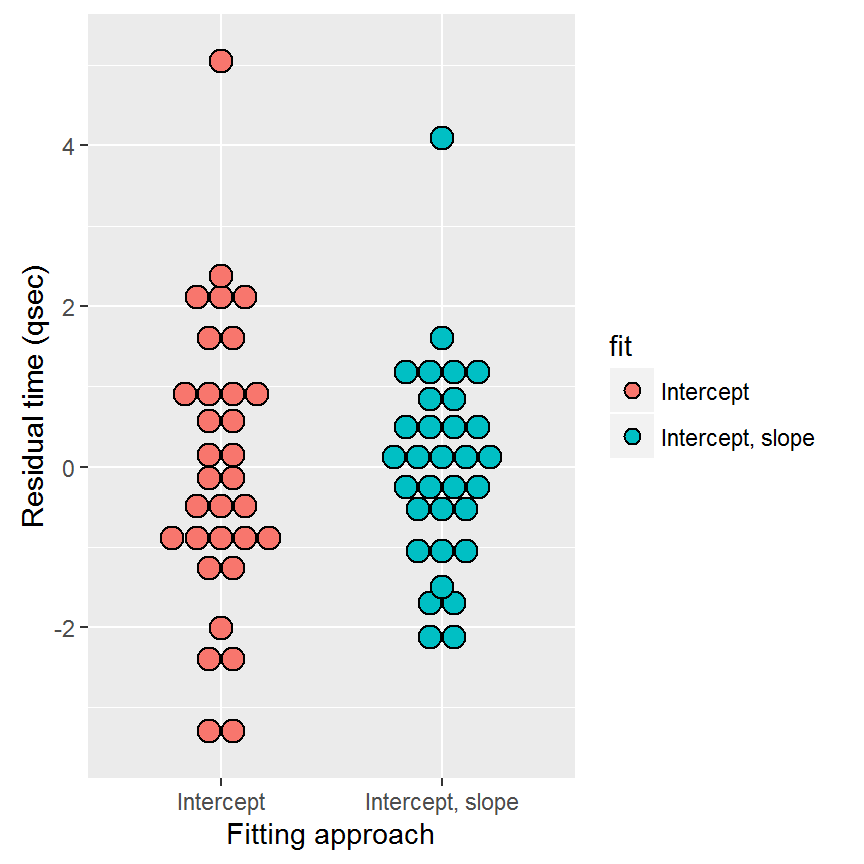In this case, the slope does not minimize the residuals substantially.

## Checking the conditions

### Conditions basics

• linearity of the relationship between the explanatory and the response variable: check by using a scatterplot or a residuals plot of the data.

• normality of the residuals: check using a histogram or a normal probability plot of the residuals.

• homoscedasticity or constant variability of the residuals. This means that the residuals should vary homogeneously around the 0 line.

Before making a predicition, check also whether the prediction takes place in the realm of the data (or whether it is probably an extrapolation).

https://gallery.shinyapps.io/slr_diag/

### Graphical tools for regression diagnostics

The Summary of the fit

``````xvar <- mtcars\$hp #input explanatory variable
yvar <- mtcars\$qsec #input response variable
mydata <- cbind(xvar,yvar)
mydata <- data.frame(mydata) #produce data.frame as required by ggplot
testfit <- lm(yvar ~ xvar) #fit the data (response as explained by explanatory variable)
summary(testfit) #show summary``````
``````##
## Call:
## lm(formula = yvar ~ xvar)
##
## Residuals:
##     Min      1Q  Median      3Q     Max
## -2.1766 -0.6975  0.0348  0.6520  4.0972
##
## Coefficients:
##              Estimate Std. Error t value Pr(>|t|)
## (Intercept) 20.556354   0.542424  37.897  < 2e-16 ***
## xvar        -0.018458   0.003359  -5.495 5.77e-06 ***
## ---
## Signif. codes:  0 '***' 0.001 '**' 0.01 '*' 0.05 '.' 0.1 ' ' 1
##
## Residual standard error: 1.282 on 30 degrees of freedom
## Multiple R-squared:  0.5016, Adjusted R-squared:  0.485
## F-statistic: 30.19 on 1 and 30 DF,  p-value: 5.766e-06``````
• Check the P-value: small enough?

• Check the R-squared: high enough?

• What about the slope far enough from 0?

Regression plot

``````# SCATTERPLOT (in this case geom_smooth, because values are not float)
par(mfrow=c(1,1))
g <- ggplot(data=mydata,aes(x=xvar,y=yvar)) +
geom_point() +
geom_smooth() +
labs(title="1/2 mile time as explained by gross horsepower") +
labs(y="1/2 mile time",x="horsepower") +
theme(plot.title = element_text(family = "sans", color="#084B8A", face="bold", size=14, hjust=0.5)) +
geom_abline(slope=testfit\$coefficients,intercept=testfit\$coefficients,col="#0000CC")
g``````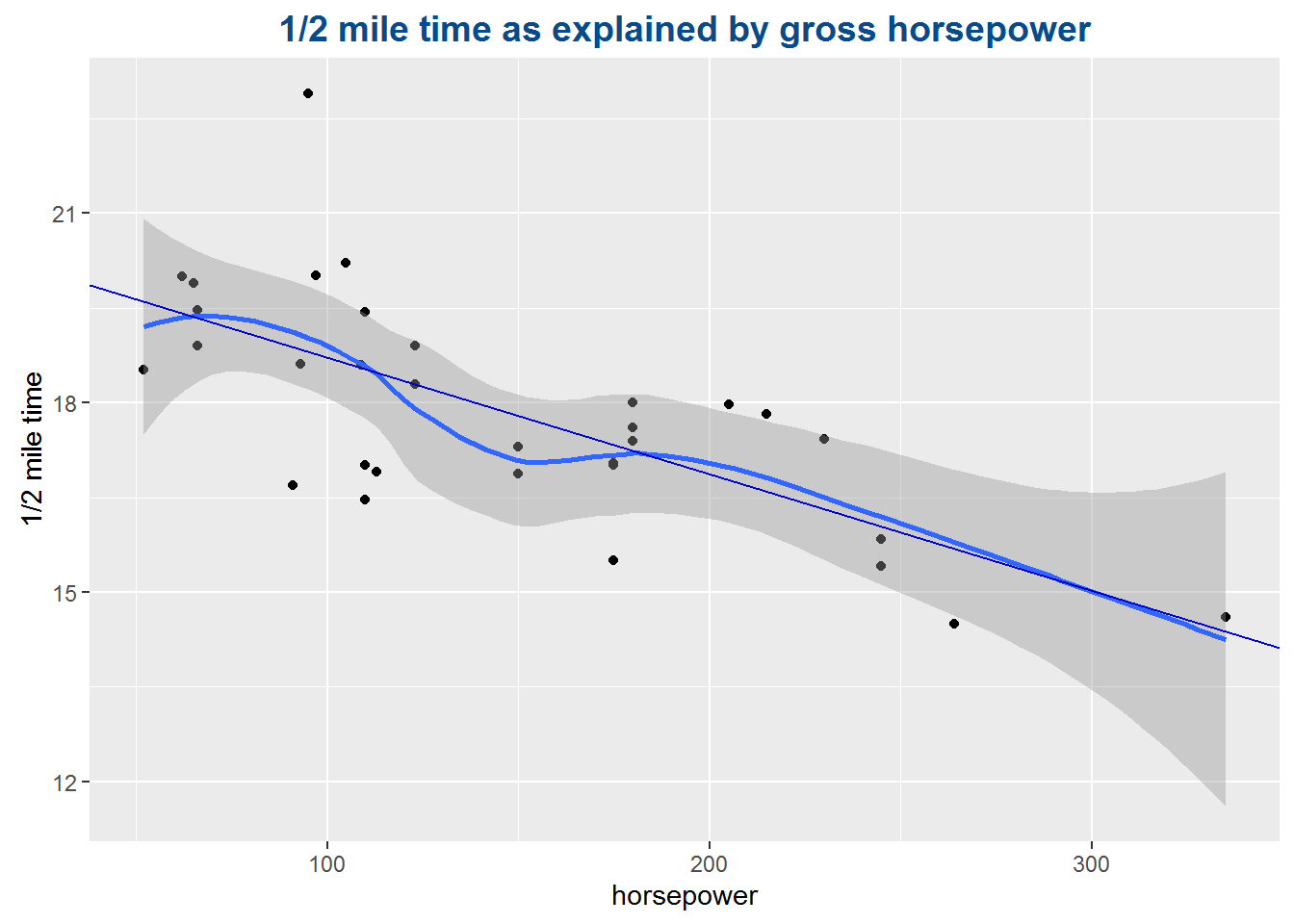``par(mfrow=c(1,3))``
• Check for scatter and linearity

Residual plot

``````mydata\$residuals <- resid(testfit)
ggplot(data = mydata, aes(x = xvar, y = residuals, colour=abs(residuals))) +
geom_point() +
geom_hline(yintercept = 0, linetype = "dashed") +
scale_colour_gradient("Residual value", low = "#000000", high = "#FF0000") +
labs(title="Residual plot: x-values vs Residuals") +
theme(plot.title = element_text(family = "sans", color="#084B8A", face="bold", size=14, hjust=0.5)) +
xlab("x-values") +
ylab("Residuals")``````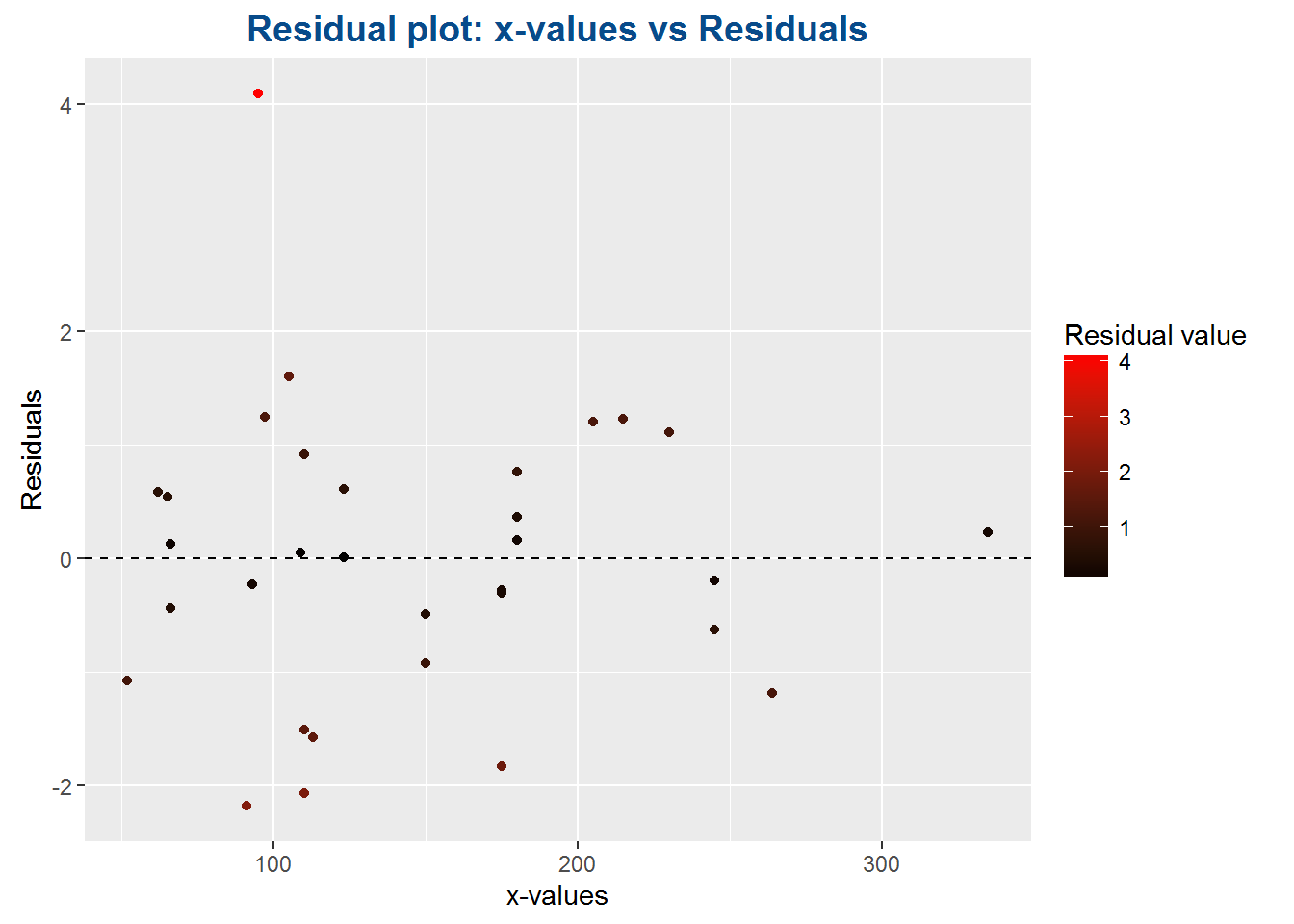``````ggplot(data = testfit, aes(x = testfit\$fitted.values, y = testfit\$residuals, colour=abs(testfit\$residuals))) +
geom_point() +
geom_hline(yintercept = 0, linetype = "dashed") +
scale_colour_gradient("Residual value", low = "#000000", high = "#FF0000") +
labs(title="Residual plot: Fitted values vs Residuals") +
theme(plot.title = element_text(family = "sans", color="#084B8A", face="bold", size=14, hjust=0.5)) +
xlab("Fitted values") +
ylab("Residuals")``````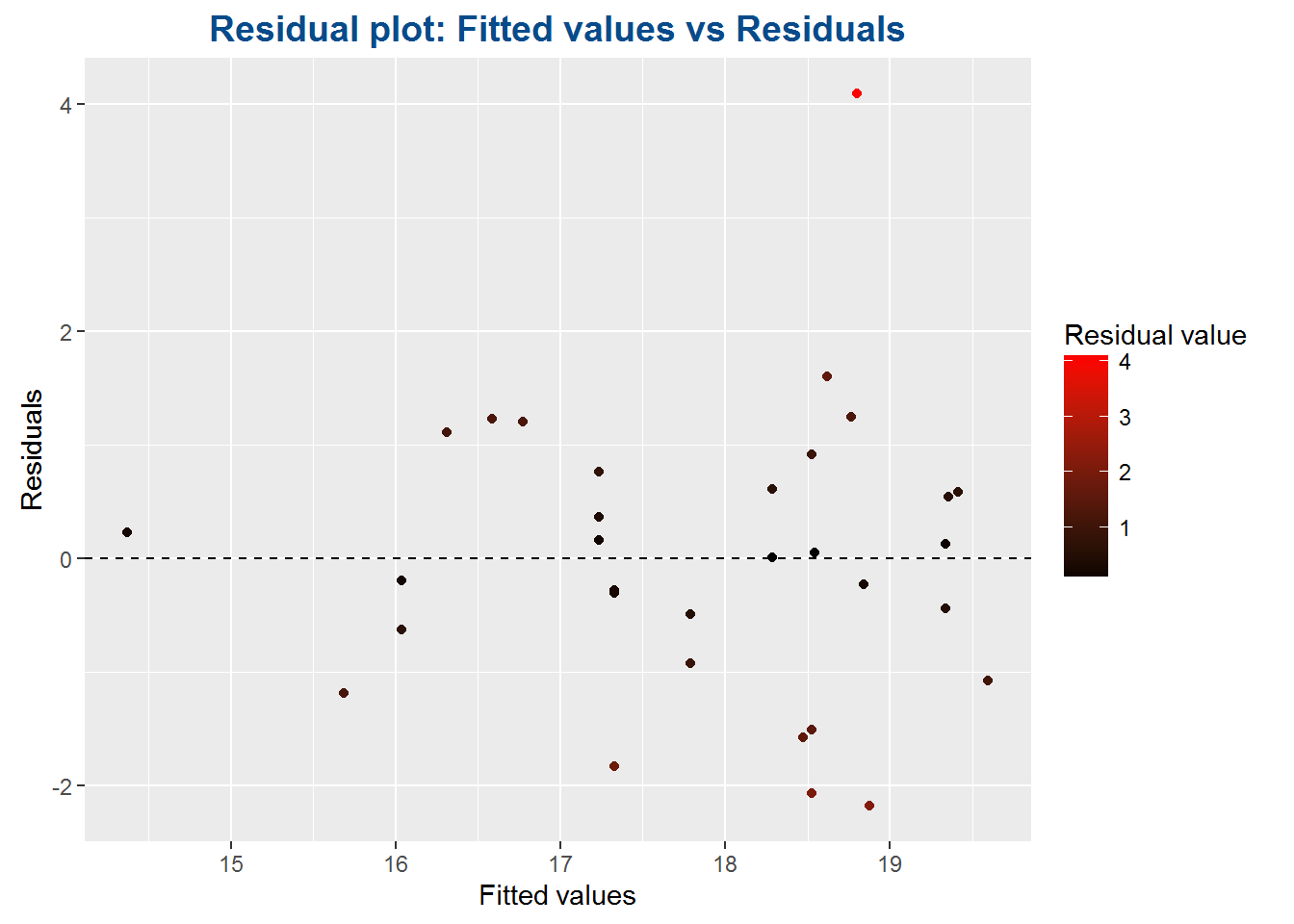The importance of producing a scatteplot with the fitted values as explanatory variable (rather than the index OR the observed values) is to show how prediction influences residuals. It shows the distance between the predicted and observed values. For instance, a model can be great at predicting values above 100, but weak at predicting values smaller than 100. This shows in which range the model performs well.

• Check for constant variability (no fan-shaped scatter)

• Check for linearity (no curve or other non-linear form)

Histogram

``````ggplot(data = testfit, aes(x = testfit\$residuals)) +
geom_histogram() +
labs(title="Distribution of residuals") +
labs(x="residuals") +
theme(plot.title = element_text(family = "sans", color="#084B8A", face="bold", size=14, hjust=0.5))``````
``## `stat_bin()` using `bins = 30`. Pick better value with `binwidth`.``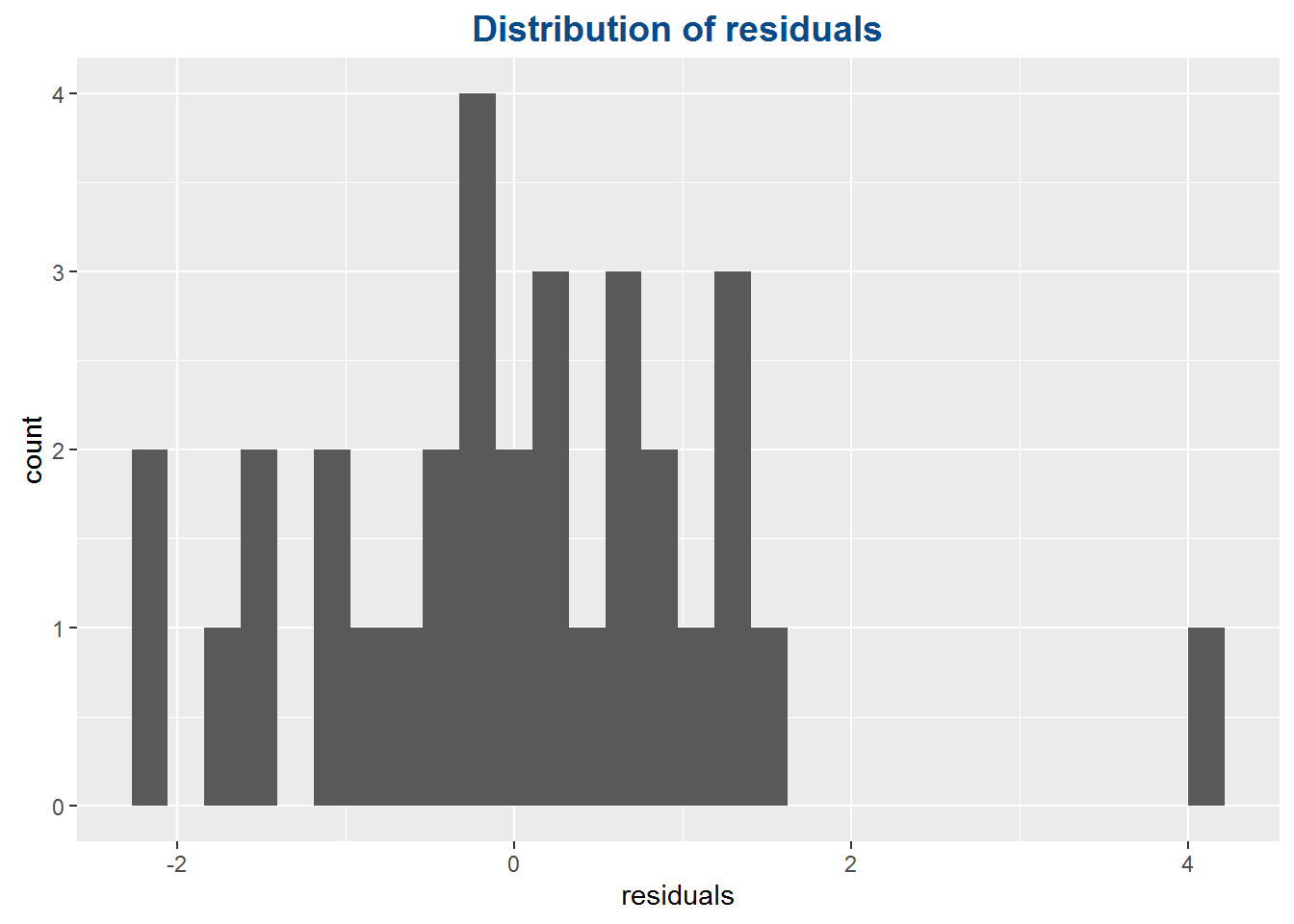• Check for normality of distribution

Normal probability plot

``````qqnorm(y=testfit\$residuals,
main="Normal probability plot",
xlab="residuals")
qqline(y=testfit\$residuals)``````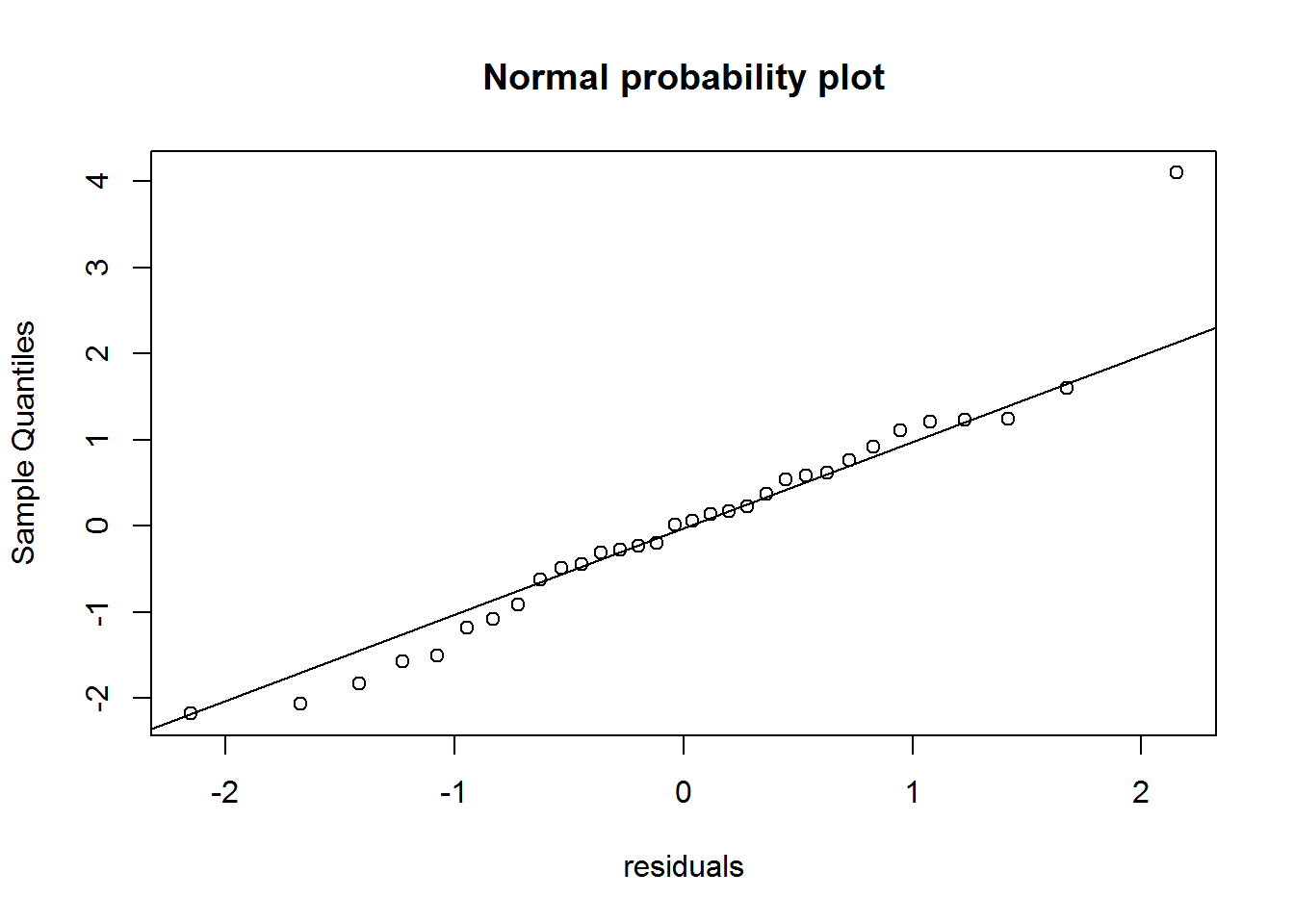Comparison: normal distribution vs residual distribution

``````plotnorm<-dnorm(testfit\$residuals,mean=mean(testfit\$residuals),sd=sd(testfit\$residuals))

plot(density(testfit\$residuals),main="Comparison: normal distribution vs residual distribution")
points(x=testfit\$residuals,y=plotnorm,col="blue",pch=16)``````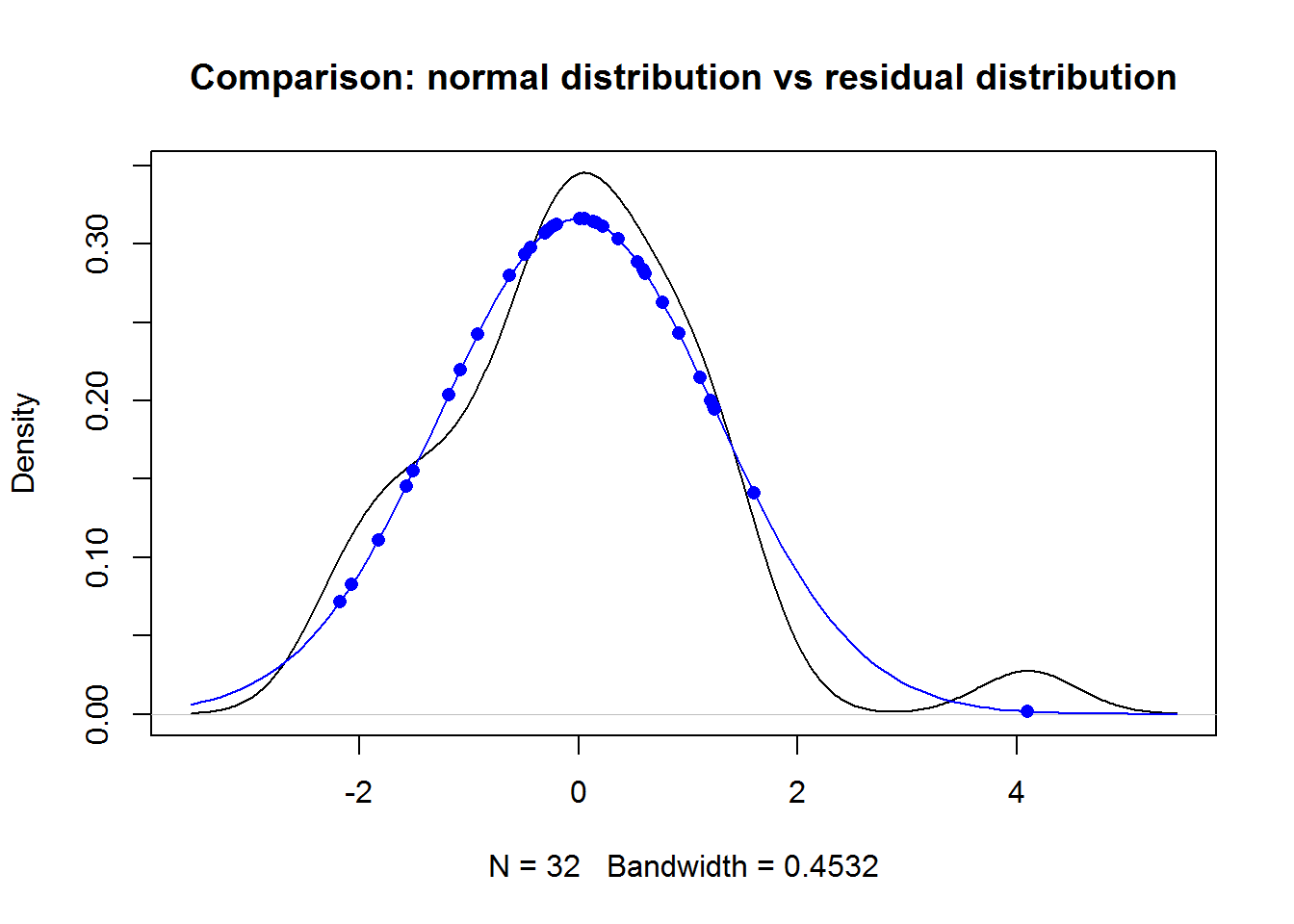Difference: normal distribution vs residual distribution

And here is my take on a detrended quantile-quantile plot to highlight the difference between the normal distribution and the distribution of residuals.

It should work better with a larger set of residuals (only 32 in this example). You can see the same pattern as in the previous two plots.

``````g <- hist(testfit\$residuals,breaks=20,plot=FALSE)
nb_newbreaks <- length(g\$breaks)-1
breaks_distance <- (max(g\$breaks)-min(g\$breaks))/(nb_newbreaks)
newbreaks_increment <- breaks_distance/2
g\$newbreaks <- g\$breaks[1:(length(g\$breaks)-1)]+newbreaks_increment
g\$norms <- dnorm(g\$newbreaks,mean=mean(testfit\$residuals),sd=sd(testfit\$residuals))
g\$diff <- g\$density-g\$norms
barplot(g\$diff,main="Difference: normal distribution vs residual distribution")``````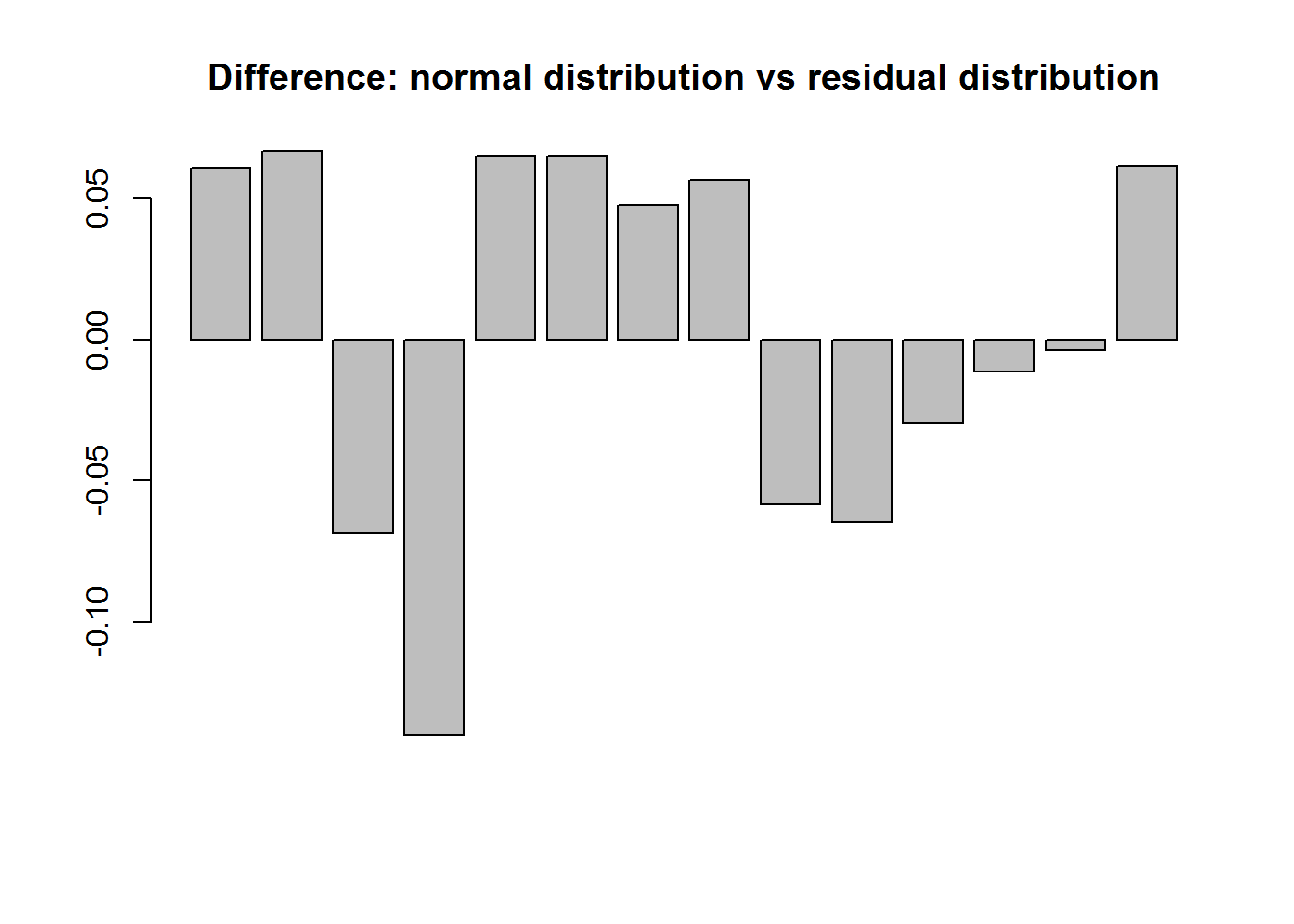• Check (again) for normality of distribution

## Evaluating the strength of the fit

Calculate R Squared (R^2), which tells us what percent of the variability is explained by the model.

The variance of the response variable minus the variance of the residuals, over the variance of the response variable is the part of variance reduced thanks to the model:

`R_squared = (sd_response^2 - sd_residuals^2)/sd_response^2`

R_squared is also expressed by ANOVA, taking the sum of squares of the response variable over the total sum of squares. Let us proceed to a manual check:

``````R_squared1 <- sum((testfit\$fitted.values - mean(testfit\$fitted.values))^2) / sum((yvar - mean(yvar))^2)
R_squared2 <- cor(x=xvar,y=yvar)^2
round(R_squared1,4)==round(R_squared2,4)``````
``##  TRUE``

### Calculating variance “by hand”

Total sum of squares: the sum of squared distances between x and the mean of x

• `sTot <- sum((x-mean(x))^2)`

The sum of squared residuals (unexplained by the model)

• `sRes <- deviance(fit)`

The variability explained by the model:

• `sExp <- sTot - sRes`

The part of variability explained by the model:

• `R_squared <- 1-sRes/sTot`

OR

• `R_squared <- sExp/sTot`

# Regression with categorical explanatory variables

## Categorical predictors with two levels

The explanatory variable is set as factors with values 0 and 1 (e.g. 0 for “automatic transmission”, 1 for “manual” in the `mtcars` dataset).

Whereas the intercept equals the mean of the first category of values (“used games”), the slope expressed the increase from the mean of one category to the other.

# Prediction

### Confidence interval != prediction interval

Confidence interval: 95% sure line is between x_1 and x_2 (used for the line)

• `sqrt((1/n) + ((x0 - X_bar)^2 / sum((X_i - X_bar)^2) ) )`

Prediction interval: 95 points are between x_3 and x_4 (used for ys)

• `sqrt(1 + (1/n) + ((x0 - X_bar)^2 / sum((X_i - X_bar)^2) ) )`

# Inference for linear regression

## Confidence interval

`beta_1 + c(-1,1) * t_score * t_value`

t_value = (beta_1 – null_value)/SE_slope

You can find the slope (beta_1), the standard error of the slope and the t_value in the fit summary.

Do not forget to use the right degrees of freedom when calculating the t_score, also indicated in the summary.

## Hypothesis test

The Null Hypothesis is the slope is zero (hence no correlation)

The alternative hypothesis is that the slope is not zero.

`pt(q=t_value,df=df) * sides`